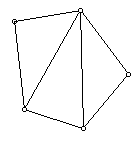# Sum It Up!

Can you create a triangle with two angles that measure 30 and 50 degrees?

How about 90 and 95 degrees?
How about 10 and 120 degrees?
How about 105 and 85 degrees?

Based on this investigation, a triangle must have what angle sum?

Devise and explain several different ways to illustrate the sum of the angles in a triangle.Extension
Extension-1:   Given the following angle in a triangle, what different types of triangles (acute, right, obtuse, scalene, isosceles, equilateral) can you form?

• Exactly one right angle
• At least one acute angle
• Exactly one obtuse angle

Extension-2:   How many non-overlapping triangles will make a quadrilateral? What is the sum of the angles in a quadrilateral?

How many non-overlapping triangles will make a pentagon? What is the sum of the angles in a pentagon?Explain the relationship between the number of sides in a convex polygon and the sum of its angles.